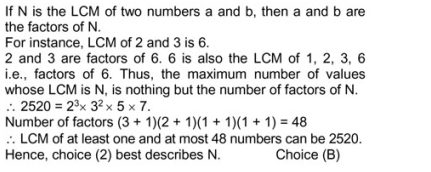# Quantitative Aptitude Quiz for SSC(Mix): 07 April 2021

Updated Wed, 07 Apr 2021 04:42 PM IST

1. What quantity of water should be mixed with 12 litres of 88% milk solution to obtain a 48% milk solution?

a) 10 litres
b) 13.6 litres
c) 14 litres
d) 8.4 litres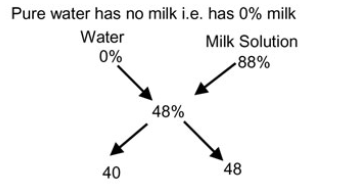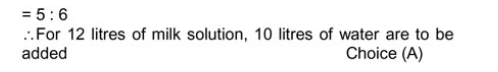2. The marked price and the cost price of a watch are in the ratio 4 : 3. The discount percentage offered before it was sold and the profit/loss percentage made on it are in the ratio 3 : 4. Find the profit/loss percentage.

a) 8 (1/3) % Loss
b) 8 (1/3) % Profit
c) 16 (2/3) % Profit
d) Cannot be determined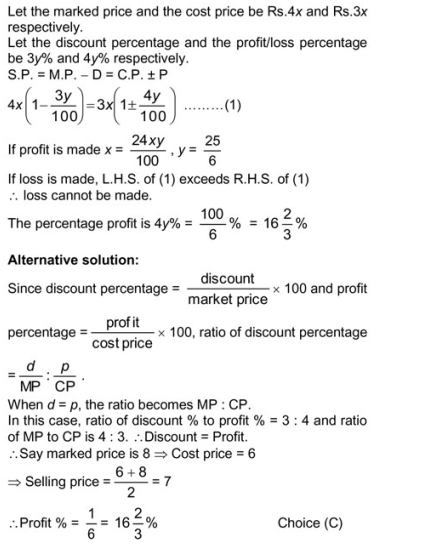3. There is a series in arithmetic progression, comprising 240 terms. The 47th term and 211th term of the progression are 23 (3/43) and 177 (13/43) respectively. To this series, P terms are added at the beginning and another (P + 17) terms are added at the end such that the entire series is still in arithmetic progression.Source: safalta

If the total number of terms in the series finally is 473, what is the sum of all the 473 terms of the series?

a) 47432
b) 44388
c) 47344
d) 47388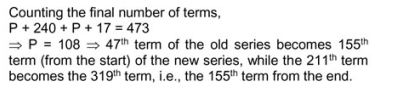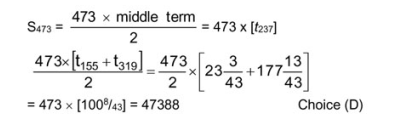4. The hands of a strange clock move such that they would meet twice as frequently if they run in opposite directions than if they run in the same direction. How many times would the faster hand meet the slower hand in the time that the slower hand completes 20 rotations, given that the hands run in opposite directions?

a) 80
b) 60
c) 40
d) 120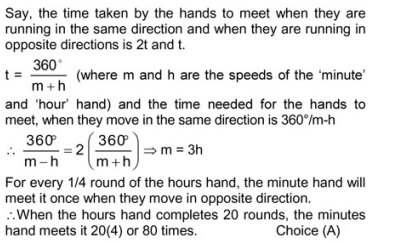5. What is the remainder when 25 × (1331)^2196 is divided by 13?

a) 11
b) 1
c) 12
d) 0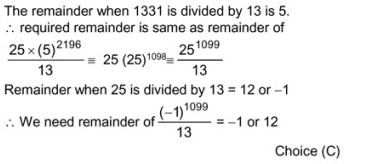6. The figure above shows two concentric squares, ABCD and EFGH, with centre O. GICJ is a square, whose area is half of that of the square EFGH.

### Free Demo Classes

If the length of the line segment AG is 6 cm, find the area (in sq. cm) of the square ABCD.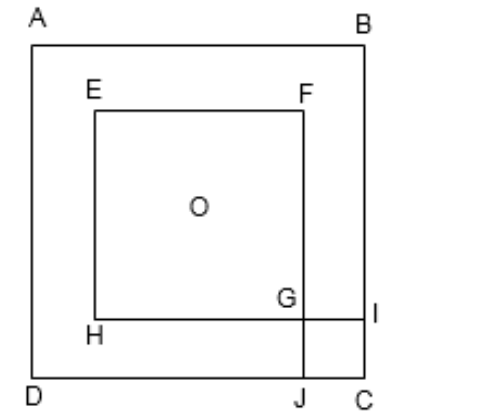a) 45
b) 72
c) 36
d) 54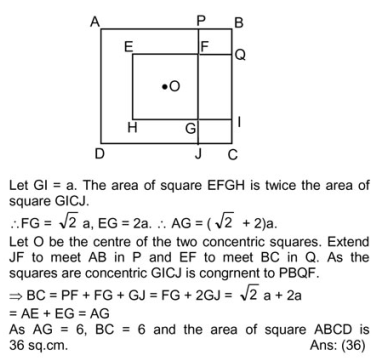7. If= 48 and pqr = 16, find the value ofa) 1
b) -2
c) 1 or -2
d) 2 or 1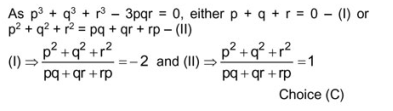8. For which of the following ranges of x is it not possible to find any value of x that satisfies the inequalitya) (-1, 1)
b) (3/2 , 5/2)
c) (7/2 , ∞)
d) None of the above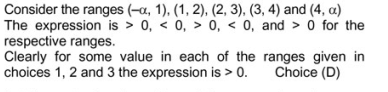9. Arjun went to a market to buy apples, bananas and oranges. He bought an equal number of bananas and oranges and twice as many apples as oranges, thereby spending all the money he had with him. The sum of the prices of 1 apple, 1 banana and 1 orange is Rs.12. Instead, if the cost of each orange was equal to the actual price of an apple, the other prices remaining the same, he would have just been able to buy as many apples and oranges that he actually bought but would have had no money left over to buy any bananas. If he purchased 60 fruits on the whole, find the expenditure he incurred on apples.

a) Rs.180
b) Rs.240
c) Rs.60
d) Rs.120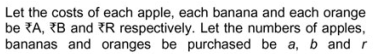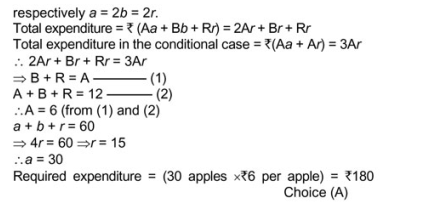10. The least common multiple of N distinct natural numbers is 2520. Which of the following best describes N?

a) 1 ≤ N ≤ 10
b) 1 ≤ N < 50
c) 1 < N < 24
d) 1 < N ≤ 40